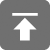# 工学1号馆

home

## C安全编码–预处理

Wu Yudong    September 11, 2016     C   569

## 建议和规则

• 用内联函数或静态函数代替与函数相似的宏
• 在宏参数名两边加上括号
• 宏替换列表应该加上括号
• 应该使用typedef定义编码类型
• 不要复用标准头文件名
• 理解连接标记或执行字符串化时的宏替换
• 把头文件放在包含防护条件中
• 避免使用连续的问号
• 保证头文件名唯一
• 不要用不安全的函数替换安全函数
• 在一个do-while循环中包装多条语句的宏

• 不要通过连接创建统一字符名称
• 不要在不安全宏的参数中包含赋值、增值、减值、volatile访问或函数调用

## 用内联函数或静态函数代替与函数相似的宏

#define CUBE(x) ((x) * (x) * (x))
/*...*/
int i = 2;
int a = 81 / CUBE(++i);

inline int cube(int x)
{
return x * x *x;
}
/*...*/
int i = 2;
int a = 81 / cube(++i);

#include<stdio.h>
size_t count = 0;
#define EXEC_BUMP(func) (func(), ++count)
void g(void) {
printf("Called g, count = %zu.\n", count);
}
void aFunc(void) {
size_t count = 0;
while(count++ <10) {
EXEC_BUMP(g);
}
}
int main(void){
aFunc();
return 0;
}#include<stdio.h>
size_t count = 0;
void g(void) {
printf("Called g, count = %zu.\n", count);
}
typedef void(*exec_func)(void);
inline void exec_bump(exec_func f) {
f();
++count;
}
void aFunc(void) {
size_t count = 0;
while(count++ <10) {
exec_bump(g);
}
}
int main(void){
aFunc();
return 0;
}#define F(x) (++operations, ++calls_to_F, 2 * x)
#define G(x) (++operations, ++calls_to_G, x + 1)
/*...*/
y = F(x) + G(x);

operations变量在同一个表达式中读取并修改了2次，因此按照某种顺序，可能会接收到错误的值

inline int f(int x) {
++operations;
++calls_to_f;
return 2 * x;
}
inline int g(int x) {
++operations;
++calls_to_f;
return 1 + x;
}
/*...*/
y = f(x) + g(x);

## 在宏参数名两边加上括号

#define CUBE(I) (I * I * I)
int a = 81 / CUBE(2 + 1)

#define CUBE(I) ((I) * (I) * (I))
int a = 81 / CUBE(2 + 1)

#define FOO(a, b, c)   bar(a, b, c)
/*...*/
FOO(arg1, arg2, arg3);

## 宏替换列表应该加上括号

#define CUBE(X) (X) * (X) * (X)
int i = 3;
int a = 81 / CUBE(i);
//被展开为： int a = 81 / i * i * i

#define CUBE(X) ((X) * (X) * (X))
int i = 3;
int a = 81 / CUBE(i);

## 应该使用typedef定义编码类型

#define cstring char *
cstring s1, s2;

typedef char * cstring;
cstring s1, s2;

## 把头文件放在包含防护条件中

#ifndef HEADER_H
#endif

## 避免使用连续的问号

 ??= # ??) ] ??! | ??( [ ??’ ^ ??> } ??/ \ ??< { ??- ~

//what is the value of a now ??/
a++;

//what is the value of a now? ?/
a++;

## 保证头文件名唯一

• 文件名中只有前8个字符保证是唯一的
• 文件名中的点号后面只有1个非数字字符
• 文件名中字符的大小写并不保证是区分的

#include<stdio.h>
#include “Library.h”
#include "library.h"
#include "utilities_math.h"
#include "utilities_physics.h"
#include "my_library.h"

Library.h和library.h可能表示同一个文件，并不清楚utilities_math和utilities_physics能否进行区分

#include<stdio.h>
#include “Lib_main.h”
#include "lib_2.h"
#include "util_math.h"
#include "util_physics.h"
#include "my_library.h"

## 不要用不安全的函数替换安全函数

#define vsnprintf(buf, size, fmt, list) \
vsprintf(buf, fmt, list)

vsprintf函数并不会检查边界，因此size参数将被丢弃，在使用不信任的数据的时候可能会导致潜在的缓冲区溢出问题

#include<stdio.h>
#ifndef __USE_ISOC99
/* 重新实现 vsnprintf()*/
#include "my_stdio.h"
#endif

## 参考资料

《C安全编码标准》